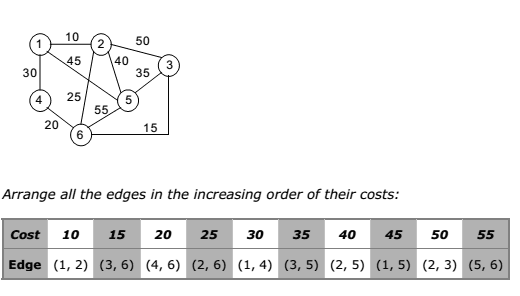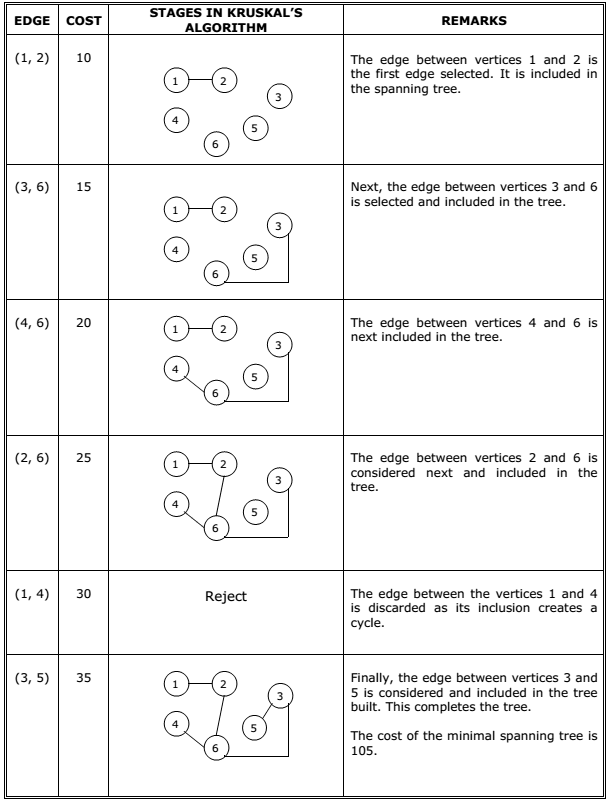This is a greedy algorithm. A greedy algorithm chooses some local optimum (i.e.
picking an edge with the least weight in a MST).

Kruskal’s algorithm works as follows: Take a graph with ‘n’ vertices, keep on adding the
shortest (least cost) edge, while avoiding the creation of cycles, until (n – 1) edges
have been added. Sometimes two or more edges may have the same cost.

The order in which the edges are chosen, in this case, does not matter. Different MST’s
may result, but they will all have the same total cost, which will always be the
minimum cost.

Kruskal’s Algorithm for minimal spanning tree is as follows:
1. Make the tree T empty.
2. Repeat the steps 3, 4 and 5 as long as T contains less than n – 1 edges and E is
not empty otherwise, proceed to step 6.
3. Choose an edge (v, w) from E of lowest cost.
4. Delete (v, w) from E.
5. If (v, w) does not create a cycle in T
6. If T contains fewer than n – 1 edges then print no spanning tree.

Example :
Construct the minimal spanning tree for the graph shown below:The stages in Kruskal’s algorithm for minimal spanning tree is as follows:You may be interested in:
Data Structures and Algorithms – MCQs.
Data Structures and Algorithms Online Tests